# Calculus 1 : Length of Line

## Example Questions

### Example Question #1 : How To Find Length Of Line By Graphing Functions

Consider a functionwith first-derivative: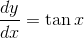.

Which integral can calculate the length along this curve betweenand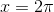?

This is not possible with the information given.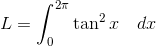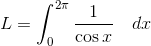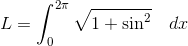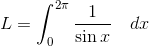Explanation:

To determine the length of a curve between two points, we evaluate the integral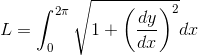There are many reasons this works, but we'll give an informal explanation here: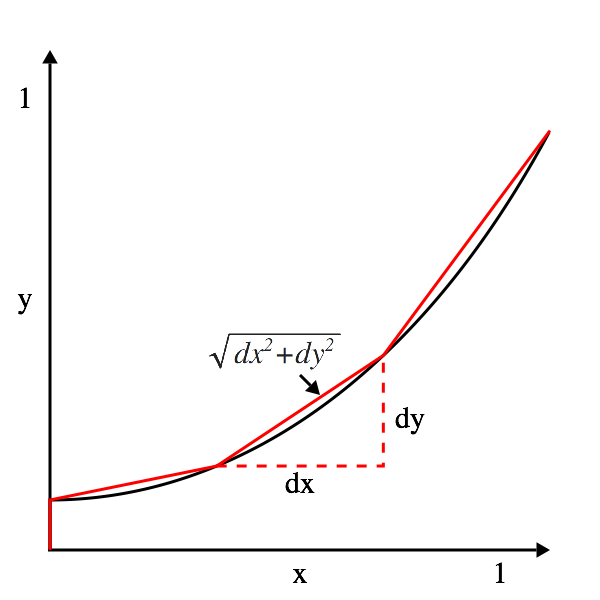If we divide this curve into three line-segments, we can see that they become more and more similar to the original curve. By adding up all the little hypotenuses, we can arrive at the length of the curve. Note, that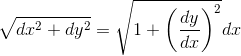If we think of the integration symbol as a sum of infinitely small parts, this gets us the formula for length: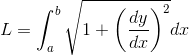.

Returning to the problem, we plug the derivative into the length formula: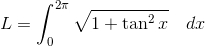substitute: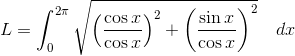Simplify: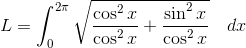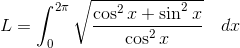By trigonometric identities we get: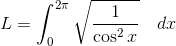###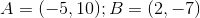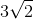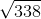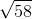Explanation:

The distance between two points can be easily found using the Distance Formula: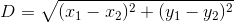Applying the points we are given to this formula results in: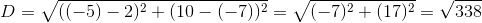This is one of the answer choices.

### All Calculus 1 Resources